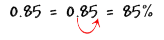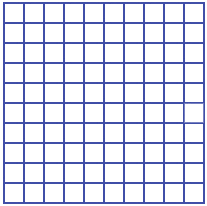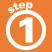Home    |    Teacher    |    Parents    |    Glossary    |    About UsPercent means "out of 100." We can use the percent symbol (%) as a handy way to write a fraction with a common denominator of 100. For example, instead of saying "8 out of every 100 professional basketball players are female," we can say "8% of professional basketball players are female."

A percent can always be written as a decimal, and a decimal can be written as a percent, by moving the decimal point two places to the right like this:The grid below has 100 squares. Use the buttons to see different percents of the grid.Homework Help | Pre-Algebra | NumbersEmail this page to a friendSearch·  Place value·  Decimal numbers·  Estimating and     rounding·  Adding / subtracting     decimals·  Multiplying decimals·  Dividing decimals·  Percent·  Exponents·  Square roots·  Signed integers·  Adding and     subtracting integers·  Multiplying and     dividing integers·  Properties of integersFirst Glance In Depth Examples WorkoutPercent